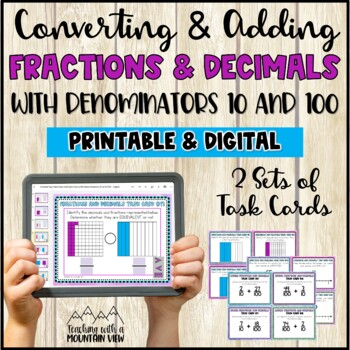# Decimals and Fractions Task Cards4th - 5th
Subjects
Standards
Resource Type
Formats Included
• Zip
•Google Apps™
Pages
23 pages
\$3.19
List Price:
\$3.99
You Save:
\$0.80
\$3.19
List Price:
\$3.99
You Save:
\$0.80The Teacher-Author indicated this resource includes assets from Google Workspace (e.g. docs, slides, etc.).

### Description

This DOUBLE set of digital and printable decimal and fractions task card activities covers converting and adding fractions and decimals with denominators of 10 and 100.

What's included in this Decimals and Fractions task card set?

• Printable Set #1: 36 Task Cards. Students identify decimals based on models and convert them to fractions. This set also includes placing a decimal/fraction on number lines and word problems. Skill Objective: Use decimal notation for fractions with denominators 10 or 100.
• Printable Set #2: 32 Task Cards. Students add fractions using equations, models, and word problems. All fractions have denominators of 10 or 100. Skill Objective: Express a fraction with denominator 10 as an equivalent fraction with denominator 100, and use this technique to add two fractions with respective denominators 10 and 100.
• A DIGITAL, Google Slides, version (of both sets!) is also included and is perfect for paperless practice and virtual learning.

These task cards are fully aligned to Common Core Standards and PERFECT for any 4th grade or older classroom!

CCSS.MATH.CONTENT.4.NF.C.5

CCSS.MATH.CONTENT.4.NF.C.6

Answer Keys and Recording Sheets Included

How can I use these Decimals and Fractions Task Cards in my classroom?

• As a pre-test or post-test activity
• As an enrichment activity
• For early finishers
• To extend learning
• As math centers
• Homework
• Differentiated math practice
• As a whole class-activity

See even more task card ideas at TaskCards.com (A Teaching With a Mountain View website)

More Fraction Resources!

Fraction Operations Error Analysis

Fractions Math Project

Total Pages
23 pages
N/A
Teaching Duration
N/A
Report this Resource to TpT
Reported resources will be reviewed by our team. Report this resource to let us know if this resource violates TpT’s content guidelines.

### Standards

to see state-specific standards (only available in the US).
Use decimal notation for fractions with denominators 10 or 100. For example, rewrite 0.62 as 62/100; describe a length as 0.62 meters; locate 0.62 on a number line diagram.
Express a fraction with denominator 10 as an equivalent fraction with denominator 100, and use this technique to add two fractions with respective denominators 10 and 100. For example, express 3/10 as 30/100, and add 3/10 + 4/100 = 34/100.